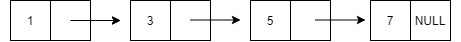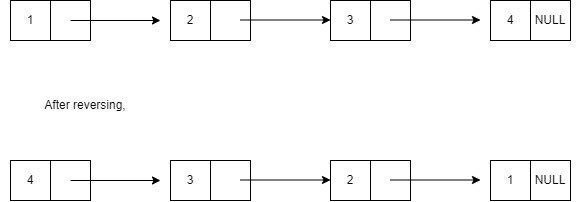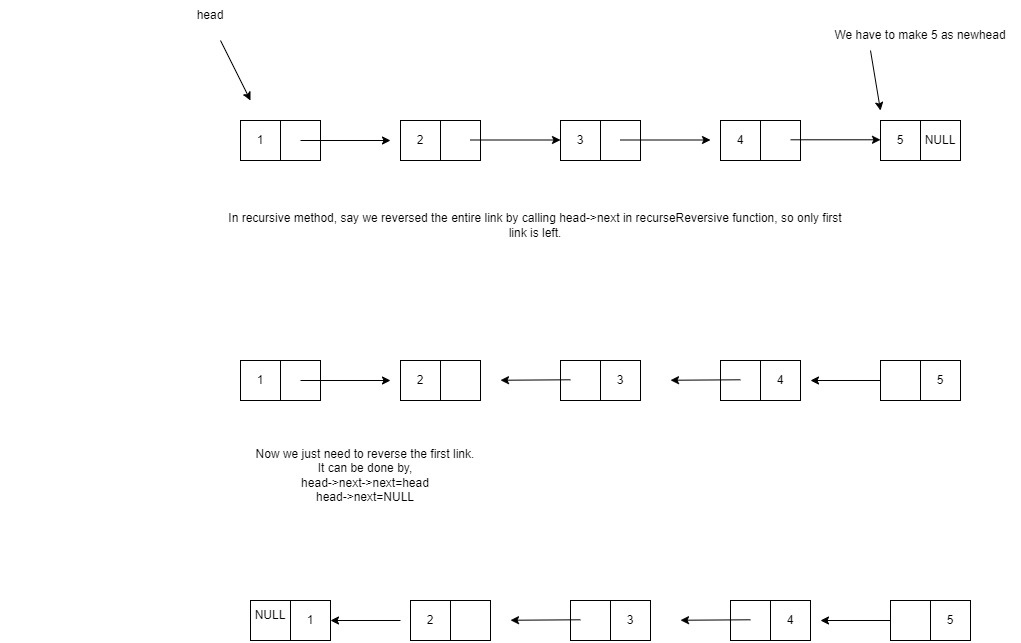# Reverse a Linked List recursively

## Introduction

In this blog, we will discuss a linked list problem that has been asked frequently in Interviews.

The problem is to Recursively reverse a Linked List which is again an important problem of LinkedList data structure.

So here I am, starting with what is a linked list?

It is an ordered set of elements, each holding data and link containing the address to the next element.Now, coming to the question.

### Problem Statement

We are given N elements in a linked list, and we are asked to reverse the elements of a linked list by using recursion.

### Sample Example

Example:## Approach

Now we will discuss the approach to reverse a linked list recursively.

Declare the Node structure and write the function to insert a node at the tail so that our linked list becomes ready.

Now to recursively reverse the linked list, first write the base condition, i.e., if only one node or no node exists.

Then call the recursive function on the entire list except for the first node.

Update the link on the first node. Return the updated list.

Time Complexity = O(n).

Since in the worst case, only one traversal is needed.Till now, I assume you must have got the basic idea of what has been asked in the problem statement. So, I strongly recommend you first give it a try.

And solve some related problems on the linked list problem

### PseudoCode

Algorithm

``````___________________________________________________________________
___________________________________________________________________
end procedure``````

## Implementation in C++

``````#include <iostream>
using namespace std;

//structure of node
class node {
public:
int data;
node* next;

node(int val){
data=val;
next=NULL;
}
};

//fuction to insert a node at the tail
void insertAtTail(node* &head, int val) {
node* n = new node(val);

return;
}

while(temp->next!=NULL) {
temp=temp->next;
}
temp->next = n;
}

//Display function
while(temp!=NULL) {
cout<<temp->data<<"->";
temp=temp->next;
}
cout<<"NULL"<<endl;
}

//function to reverse a node

//Base condition
}

}

int main() {

return 0;
}``````

Output:

``````Input:  1->2->3->4->5->NULL
Output:  5->4->3->2->1->NULL``````

### Complexity Analysis

Time Complexity: O(n).

In the worst case, all elements are to be traversed once.

∴O(n).

Space complexity: O(n) due to the space consumed by the recursive call stack

#### What is a node?

The collection of the nodes makes a linked list. The node contains the data and the address of the next node.

#### Is it possible to reverse a linked list linearly?

Yes, it is possible to reverse a linked list linear.

#### Is it necessary to initialize the last node address as null?

Yes, as elements are not stored in a contiguous manner, pointers are used, so sometimes it may take the garbage value.

## Conclusion

This article taught us how to Recursively reverse a Linked List. We discussed its implementation using illustrations, pseudocode, and then proper code.

We hope you could take away critical techniques like analyzing problems by walking over the execution of the examples and finding out the recursive pattern followed in most linked list problems.

Now, we recommend you practice problem sets based on the linked list to master your fundamentals. You can get a wide range of questions similar to this on CodeStudio

It's not the end. Must solve the problem of similar types.

Happy Coding!!